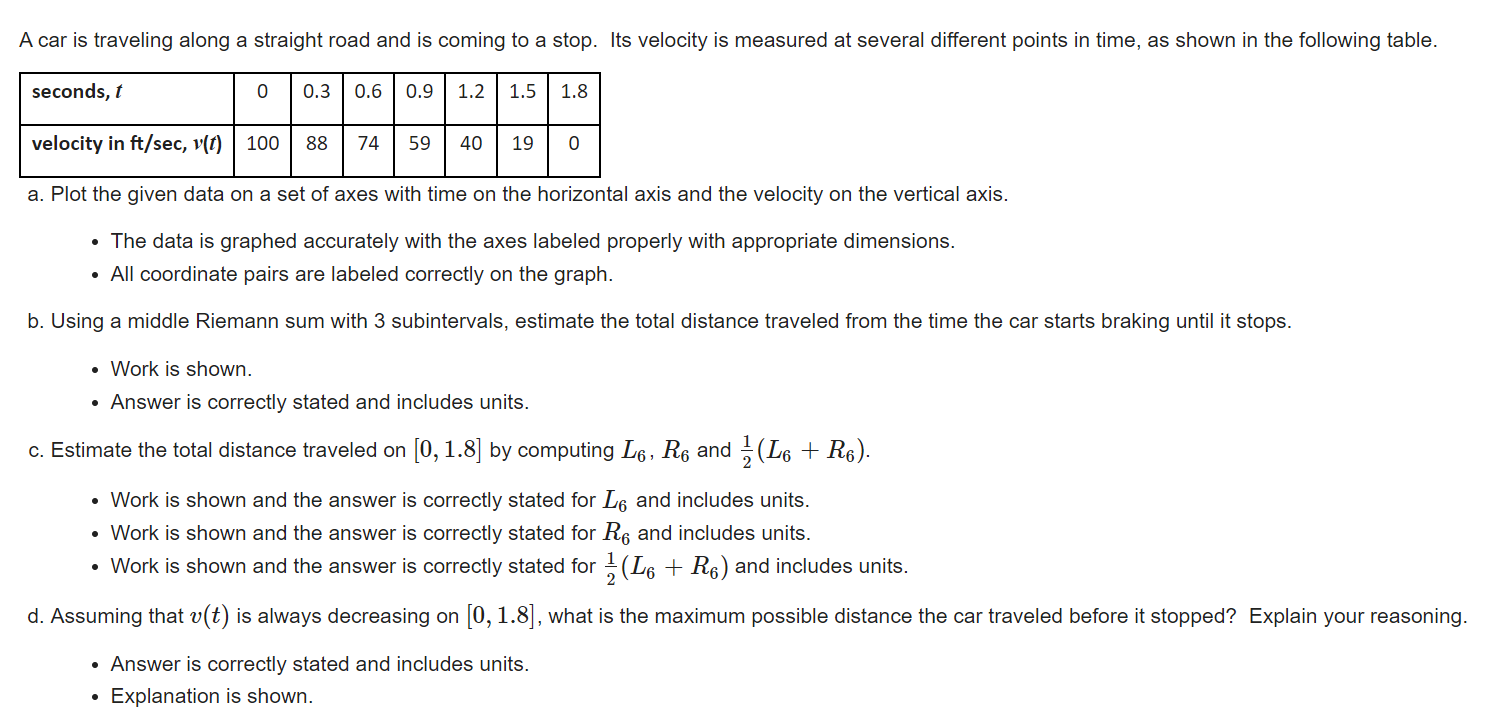# (Solved): A car is traveling along a straight road and is coming to a stop. Its velocity is measured at seve ...A car is traveling along a straight road and is coming to a stop. Its velocity is measured at several different points in time, as shown in the following table. a. Plot the given data on a set of axes with time on the horizontal axis and the velocity on the vertical axis. - The data is graphed accurately with the axes labeled properly with appropriate dimensions. - All coordinate pairs are labeled correctly on the graph. b. Using a middle Riemann sum with 3 subintervals, estimate the total distance traveled from the time the car starts braking until it stops. - Work is shown. - Answer is correctly stated and includes units. c. Estimate the total distance traveled on by computing and . - Work is shown and the answer is correctly stated for and includes units. - Work is shown and the answer is correctly stated for and includes units. - Work is shown and the answer is correctly stated for and includes units. d. Assuming that is always decreasing on , what is the maximum possible distance the car traveled before it stopped? Explain your reasoning. - Answer is correctly stated and includes units. - Explanation is shown.

We have an Answer from Expert